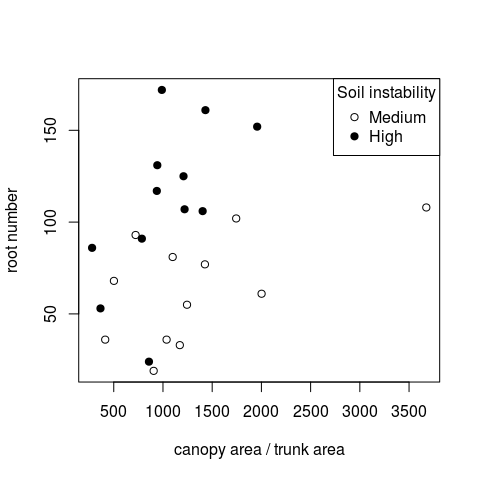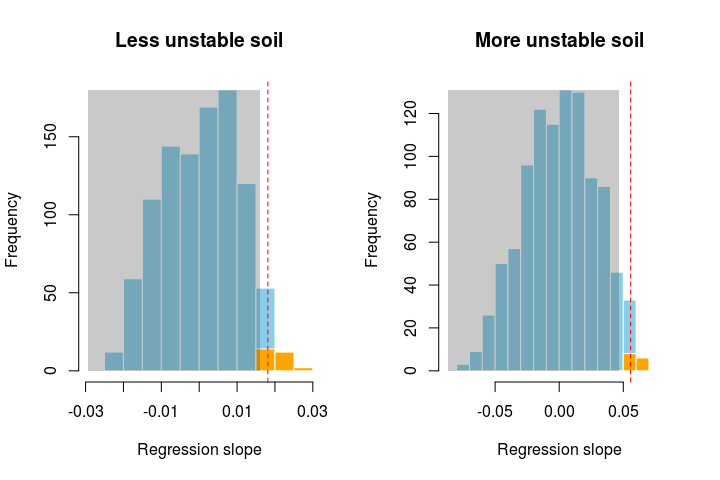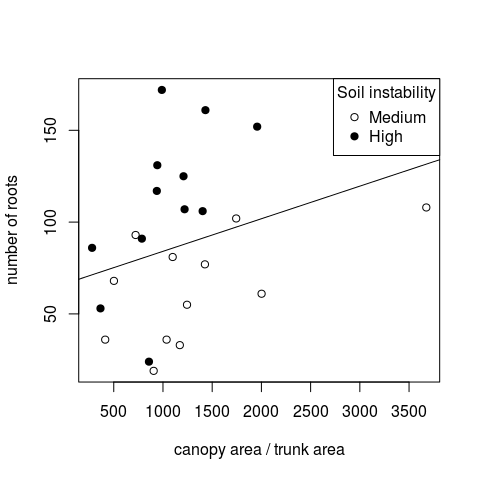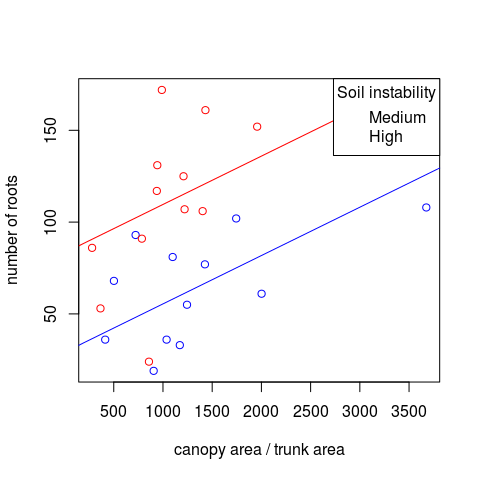Installation

Rsampling is hosted on CRAN. To install it use

> install.packages("Rsampling")

You can also install from the GitHub site, where the project is hosted. To do that use the devtools package function install_github:

> library(devtools)
> install_github(repo = 'lageIBUSP/Rsampling')

> library(Rsampling)

Regression examples

The data frame rhyzophora contains measurements of mangrove trees growing in two sites that differ in the soil stability (more or less muddy soils).

soil.instability canopy.trunk     root n.roots
1             high     937.6087 19.78200     117
2             high    1957.0434 23.66775     152
3             high    1403.0969 20.72400     106
4             high     785.6869  6.73530      91
5             high    1431.3668 15.70000     161
6             high    1208.7816 16.97170     125
> summary(rhyzophora)
soil.instability  canopy.trunk         root            n.roots
high  :12        Min.   : 279.9   Min.   : 0.5212   Min.   : 19.00
medium:12        1st Qu.: 839.7   1st Qu.: 5.9357   1st Qu.: 54.50
Median :1067.8   Median :14.7619   Median : 88.50
Mean   :1179.9   Mean   :12.7651   Mean   : 87.25
3rd Qu.:1408.9   3rd Qu.:17.3053   3rd Qu.:110.25
Max.   :3675.4   Max.   :40.5531   Max.   :172.00

Study Hypothesis

The hypothesis is that trees at more unstable soils will allocate more biomass in supporting structures. One possible prediction is that the relation between the tree’s torque 1 and the allocation in supporting roots is different in the two kinds of soils. To express the torque the ratio between the areas of the canopy and the trunk cross-section was used. The allocation in supporting roots was expressed in number of supporting roots and the area at ground level encompassed by these roots.

The data suggests a positive relation between torque and number of roots. Plus, the points of the sampled trees at the two kinds of soil seems to separate in the plot:

> plot(n.roots ~ canopy.trunk, data=rhyzophora, type="n",
+      xlab="canopy area / trunk area", ylab="root number")
> points(n.roots ~ canopy.trunk, data=rhyzophora,
+        subset=soil.instability=="medium")
> points(n.roots ~ canopy.trunk, data=rhyzophora,
+        subset=soil.instability=="high", pch=19)
> legend("topright", c("Medium","High"), title="Soil instability", pch=c(1,19))Relation between number of supporting roots and the ratio canopy area / trunk area for mangrove trees at two sites that differ in the soil instability (medium and high instability).

This pattern suggests that the relationship between torque and number of roots differ between the two sites.

Shuffling rows within the strata

Null hypothesis

In order to illustrate how to run randomization restricted to strata we will test the most basic null hypothesis: that there is no relation at none of the soil types.

Statistic of interest

We have a statistic of interest for each soil type, which is the slope of the linear regressions:

> rhyz.si <- function(dataframe){
+     m1 <- lm(n.roots ~ canopy.trunk, data=dataframe,
+              subset=soil.instability=="medium")
+     m2 <- lm(n.roots ~ canopy.trunk, data=dataframe,
+              subset=soil.instability=="high")
+     c(med = coef(m1)[],
+       high = coef(m2)[])
+ }
> ## Observed values
> rhyz.si(rhyzophora)
med       high
0.01813085 0.05570843

Distribution of the statistics under the null hypothesis

We simulate the null hypothesis shuffling the values of the torque variable between trees of the same soil type:

> rhyz.r <- Rsampling(type = "normal_rand", dataframe = rhyzophora,
+                     statistics = rhyz.si, stratum = rhyzophora$soil.instability, + cols = 2, ntrials = 1000) The argument stratum = rhyzophora$soil.instability, tells that the shuffling of values (at column 2) must be done within each soil type.

When there’s more than one statistic of interest, the function Rsampling returns a matrix where which line is a statistic and columns are the replications.

> rhyz.r[,1:3]
[,1]        [,2]         [,3]
med   0.0006732387 -0.00347208  0.001007206
high -0.0087325251  0.05450723 -0.007739720

Values which are equal or bigger than the observed slopes see very rare at the value distribution under the null hypothesis:

> par(mfrow=c(1,2))
> dplot(rhyz.r[1,], svalue=rhyz.si(rhyzophora), pside="Greater",
+       main="Less unstable soil", xlab="Regression slope")
> dplot(rhyz.r[2,], svalue=rhyz.si(rhyzophora), pside="Greater",
+       main="More unstable soil", xlab="Regression slope")Frequency distributions of the slope of linear regressions of number of supporting roots to torque in mangrove trees, under the null hypothesis that there is not a relationship. The trees were measured at two sites, and the null hypothesis was simulated shuffling y-values (n of roots) among trees at each site. Red lines show the observed values of the slopes, and the acceptance region of the null hypothesis at 5% is in grey. Absolute values large than the observed are depicted in orange. Results from 1000 simulations.

> par(mfrow=c(1,1))

Decision: should we reject the null hypothesis?

The observed slopes for the two groups are out of the region of acceptance for the one-tailed null hypothesis 2 at 5% significance level.

> sum(rhyz.r[1,] >= rhyz.si(rhyzophora))/1000 < 0.05
 TRUE
> sum(rhyz.r[2,] >= rhyz.si(rhyzophora))/1000 < 0.05
 TRUE

Conclusion: the null hypothesis is rejected (p < 0,05) at both cases.

Comparing slopes

Our main study hypothesis was that the relation between torque and support is different between the two kinds of soils. Assuming the linear relation exists, the difference can occur in two ways: different slopes or same slope but different intercepts.

Null hypothesis

We start by testing the null hypothesis that the linear the slopes of the linear regressions does not differ between soil types.

Statistic of interest

Our statistic of interest is the difference between slopes, which seems small:

> rhyz.si2 <- function(dataframe){
+     m1 <- lm(n.roots ~ canopy.trunk, data=dataframe,
+              subset=soil.instability=="medium")
+     m2 <- lm(n.roots ~ canopy.trunk, data=dataframe,
+              subset=soil.instability=="high")
+     coef(m1)[] - coef(m2)[]
+ }
>
> ## Observed values
> rhyz.si2(rhyzophora)
 -0.03757759

Null hypothesis simulation

We simulate our new null hypothesis shuffling the trees between soil types:

> rhyz.r2 <- Rsampling(type = "normal_rand", dataframe = rhyzophora,
+                     statistics = rhyz.si2,
+                         cols = 1, ntrials = 1000)

Decision: should we reject the null hypothesis?

In this case, we cannot reject the null hypothesis at the 5% significance level:

> sum(rhyz.r2 > rhyz.si2(rhyzophora))/1000 < 0.05
 FALSE

Comparing intercepts

We have decided above to accept the null hypothesis that the slopes are equal. The biological interpretation of this fact is that at both soil types the number of support roots follows the same proportionality relation with the torque variable.

This proportionality factor is the slope of the linear regressions applied to all trees, which we estimate by adjusting the regression to whole data set:

> lm(n.roots ~ canopy.trunk, data=rhyzophora)

Call:
lm(formula = n.roots ~ canopy.trunk, data = rhyzophora)

Coefficients:
(Intercept)  canopy.trunk
66.30197       0.01775

That is, to each increment of 100 units of the torque variable in average 1.8 roots are added.

This proportionality is maintained if we add any constant. For this reason the linear model is expressed by:

$E[Y] = \alpha + \beta X$

Where $$E[Y]$$ is the expected value of the response variable (root number), $$\beta$$ is the slope or proportionality factor, and $$X$$ is the predictor variable (torque). The intercept $$\alpha$$ does not change the proportionality, rather, it only moves the regression line upwards or downwards. In other words, lines with same slope but different intercepts are parallel. In our case, different intercepts with the same slope express that trees with the same canopy/trunk ratio always have more roots at one of the soil types.

Null hypothesis

Now our null hypothesis is that the intercepts of the linear regressions do not differ between soil types. If this is true, the linear regression adjusted to all data would predict well the number of roots for all trees. If not, the points of one soil type will tend to fall below the line, while the points for the other soil type will fall above it.

We already adjusted the regression for the whole data set, and then we can add the regression line to the plot:

> plot(n.roots ~ canopy.trunk, data=rhyzophora, type="n",
+      xlab="canopy area / trunk area", ylab="number of roots")
> points(n.roots ~ canopy.trunk, data=rhyzophora,
+        subset=soil.instability=="medium")
> points(n.roots ~ canopy.trunk, data=rhyzophora,
+        subset=soil.instability=="high", pch=19)
> abline(lm(n.roots ~ canopy.trunk, data=rhyzophora))
> legend("topright", c("Medium","High"), title="Soil instability", pch=c(1,19))Relation between the number of supporting roots and the ratio canopy area / trunk area for mangrove trees at two sites that differ in the soil instability (medium and high instability). Also shown the line of the linear regression fitted to the whole data set.

Indeed it seems that this regression underestimates the number of roots of the trees sampled at the site with the most unstable soil, and does the opposite for the trees at sampled the site with less unstable soil. For this reason, the residuals of this regression are positive for trees sampled at unstable soil and negative for the rest.

Statistic of interest

Our statistic of interest is the difference between the means of the residual of trees at each soil type. The residuals are calculated from the regression applied to all data:

> rhyz.si3 <- function(dataframe){
+     m1 <- lm(n.roots ~ canopy.trunk, data=dataframe)
+     res.media <- tapply(resid(m1), dataframe\$soil.instability, mean)
+     res.media[] - res.media[]
+ }
> ## Observed values
> rhyz.si3(rhyzophora)
 51.60582

Simulating the null hypothesis

We simulate the null hypothesis in the same way as before: shuffling the trees between soil types (first row of the data table)

> rhyz.r3 <- Rsampling(type = "normal_rand", dataframe = rhyzophora,
+                     statistics = rhyz.si3,
+                         cols = 1, ntrials = 1000)

Decision: should we reject the null hypothesis?

In this case we reject the null hypothesis:

> sum(rhyz.r3 > rhyz.si3(rhyzophora))/1000 < 0.05
 TRUE

Therefore, there is one intercept for each soil type. We can estimate them including the soil’s effect on the regression model 3:

> (rhyz.ancova <- lm(n.roots ~ soil.instability + canopy.trunk  -1,
+                    data=rhyzophora))

Call:
lm(formula = n.roots ~ soil.instability + canopy.trunk - 1, data = rhyzophora)

Coefficients:
soil.instabilityhigh  soil.instabilitymedium            canopy.trunk
83.25788                29.10476                 0.02633

And then we can add the lines for the two groups to the plot:

> cfs <- coef(rhyz.ancova)
> plot(n.roots ~ canopy.trunk, data=rhyzophora, type="n",
+      xlab="canopy area / trunk area", ylab="number of roots")
> points(n.roots ~ canopy.trunk, data=rhyzophora,
+        subset=soil.instability=="medium", col="blue")
> points(n.roots ~ canopy.trunk, data=rhyzophora,
+        subset=soil.instability=="high", col="red")
> abline(cfs,cfs, col="red")
> abline(cfs,cfs, col="blue")
> legend("topright", c("Medium","High"), title="Soil instability", col=c("blue", "red"))Relation between number of supporting roots and the ratio canopy area / trunk area for mangrove trees at two sites that differ in the soil instability (medium and high instability). Also shown the line of the regressions fitted to each site, but with a common slope.

1. Roughly, for our purpose the torque express the force to bring the tree down.

2. As it doesn’t make sense, in this case, to expect the number of roots to decrease with the torque variable, we did the one-tailed test.

3. Technical detail: we add the term -1 to the regression formula in order to explicit to R that we want the estimates of each intercept. Otherwise, we’d get the estimation the intercept of one group and the difference to the intercept of the other group.